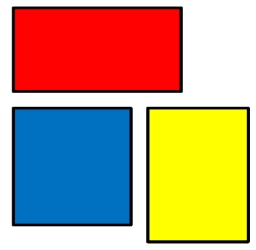# Rectangle 80701

One side of the rectangle has length a=9/5 cm, and the length of side b is 7/10 cm greater than the length of side a.
Calculate the perimeter and area of ​​the rectangle.

o =  8.6 cm
S =  4.5 cm2

### Step-by-step explanation:Did you find an error or inaccuracy? Feel free to write us. Thank you!

Tips for related online calculators
Need help calculating sum, simplifying, or multiplying fractions? Try our fraction calculator.

#### Grade of the word problem:

We encourage you to watch this tutorial video on this math problem: